红黑树

常用的二叉树类型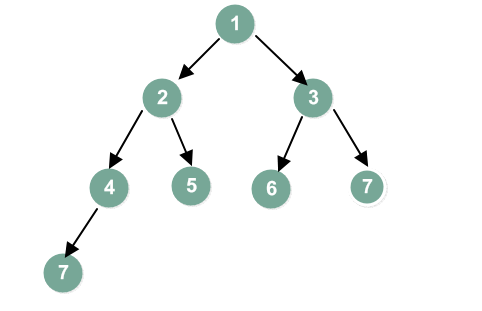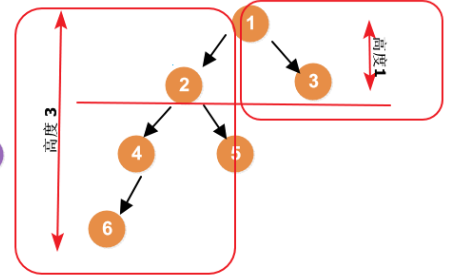。保证每个节点被访问的次数平衡。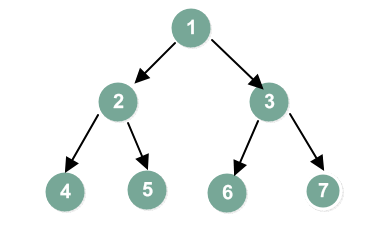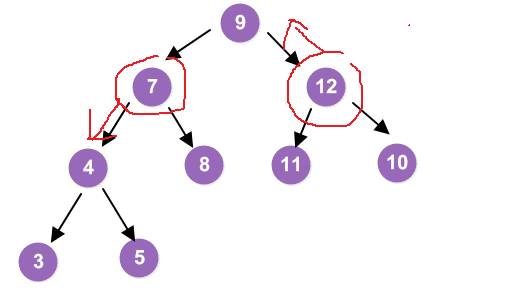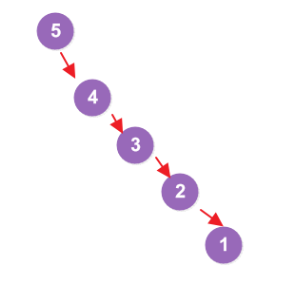• 深度定义是从上往下的
• 高度定义是从下往上的
• 空数高度0
• 叶子结点高度1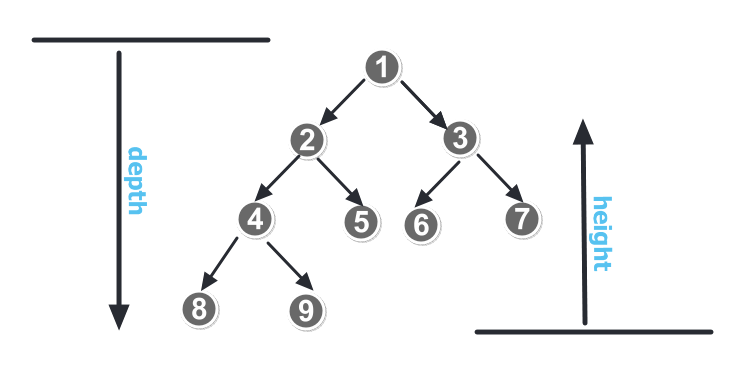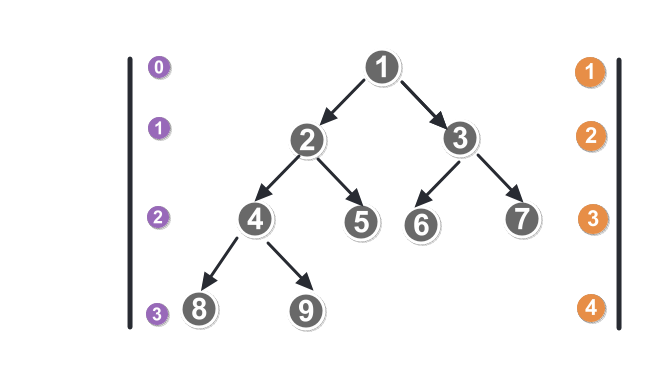•  根结点0紫色，层数=深度=高度-1
根结点1橙色，层数=深度=高度


•  相同深度的每个结点，高度不一定相同，因为每个结点下面的叶结点的深度是不一定相同的


红黑树原理

1. 每个节点要么是红色，要么是黑色。
2. 根节点必须是黑色
3. 红色节点不能连续（红色节点的孩子和父亲都不能都是红色）
4. 从任意节点出发，到其所有叶子节点的简单路径上都包含相同数目的黑色节点.（非常重要）
5. 每个红色节点的两个子节点一定都是黑色（叶子节点包含NULL）

红黑树的结构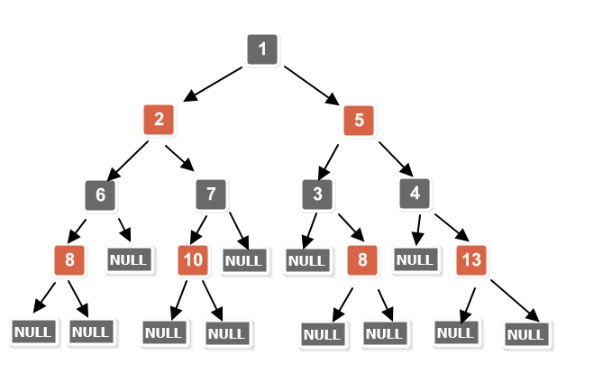红黑树插入过程中情况

1. 红黑树结构不会旋转变化情况：
1）当插入的节点为的父亲为黑色节点。【什么都不用做】
2）被插入的节点是根节点。【直接把此节点涂为黑色】
2. 红黑树结构发生旋转变化情况：
1） 当前节点的父节点【60】是红色，且当前节点的祖父节点【40】的另一个子节点（叔叔节点）也是红色。
2）当前插入的父节点是红色，当前叔叔节点的黑色，且当前节点为其父亲节点的左孩子。（进行左旋）
3）当前插入的父节点是红色，当前叔叔节点的黑色，且当前节点为其父亲节点的右孩子。（进行右旋）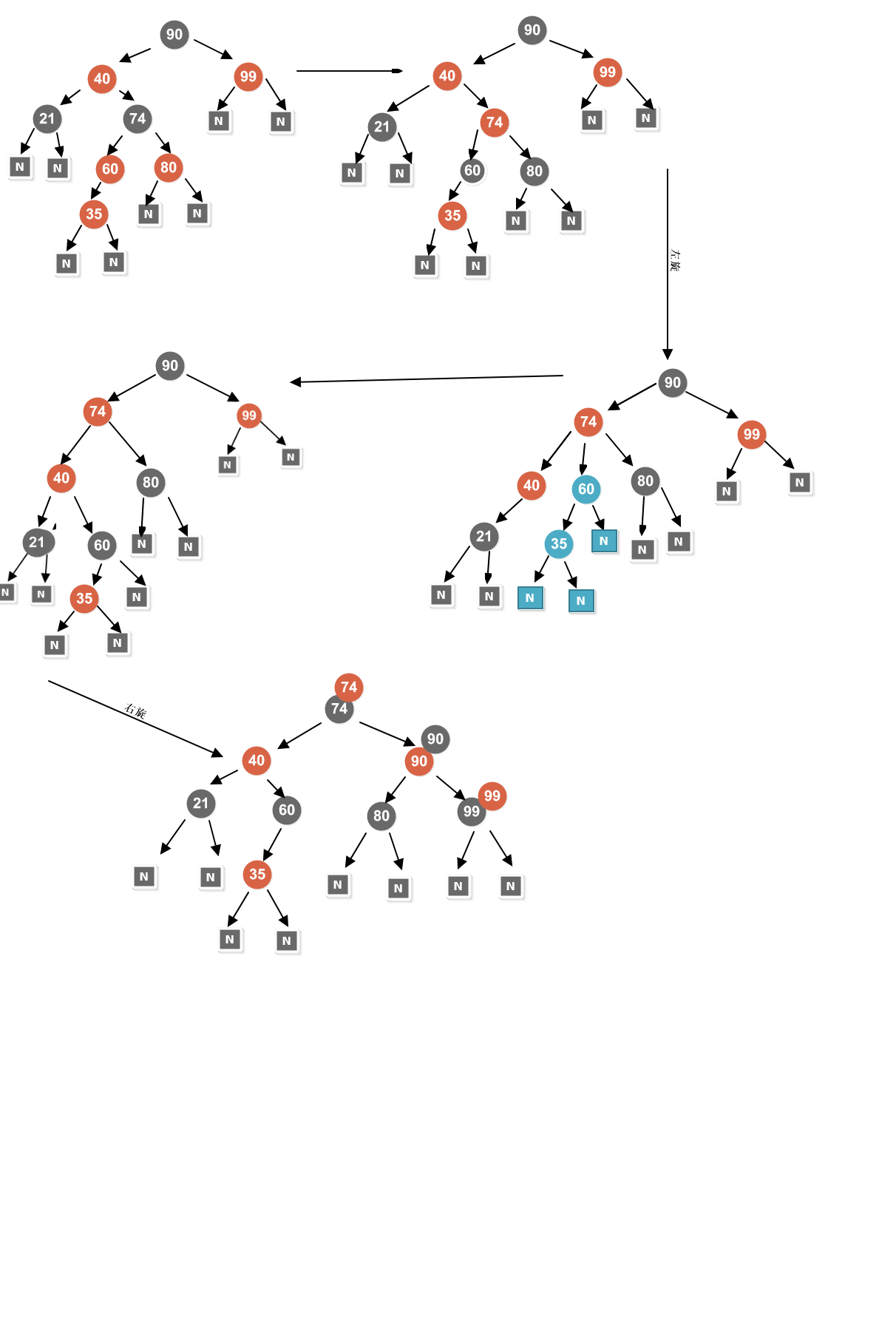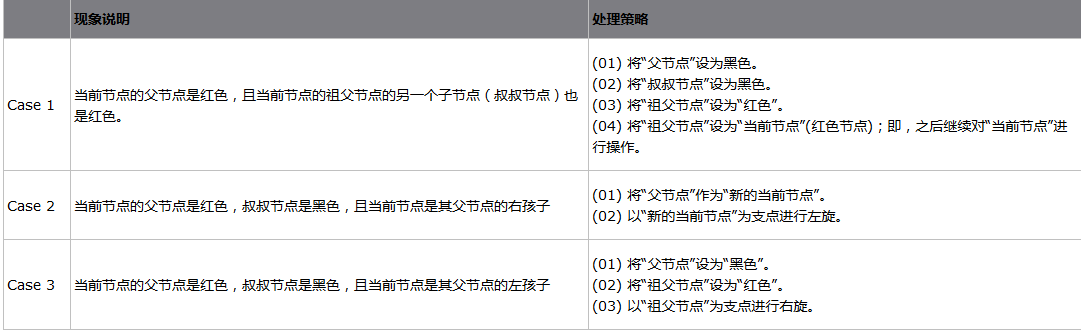树的旋转问题

1.为什么会出现旋转？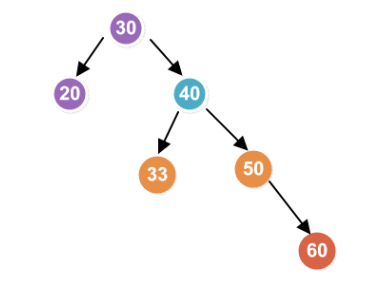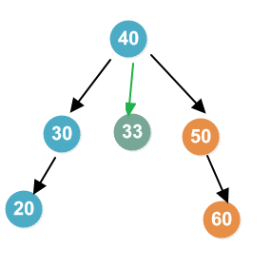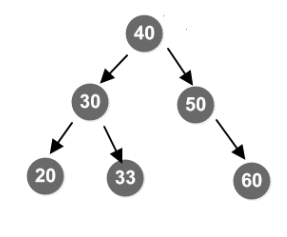红黑树应用

TreeMap 典型红黑树
Android Binder 虚拟内存 Intent IPC 1M 小内存块
TreeMap

//左旋右侧需要平衡
private void rotateLeft(Entry<K,V> p) {
if (p != null) {
//拿到根节点的右子节点
Entry<K,V> r = p.right;
//把根节点的右子节点的左节点，赋值
p.right = r.left;
if (r.left != null)
//将根节点这个值赋值到当前断开的跟节点上
r.left.parent = p;
//r 将来要成为新的根节点 p.parent 为根 ，使得他为新的跟节点
r.parent = p.parent;
if (p.parent == null)
root = r;
//如果p 为左孩子，让他还是成为左孩子 同理
else if (p.parent.left == p)
p.parent.left = r;
else
p.parent.right = r;
//最后 将当前交换的跟换值
r.left = p;
p.parent = r;
}
}


 private void rotateRight(Entry<K,V> p) {
if (p != null) {
Entry<K,V> l = p.left;
p.left = l.right;
if (l.right != null) l.right.parent = p;
l.parent = p.parent;
if (p.parent == null)
root = l;
else if (p.parent.right == p)
p.parent.right = l;
else p.parent.left = l;
l.right = p;
p.parent = l;
}
}


  public V put(K key, V value) {
Entry<K,V> t = root;
if (t == null) {
compare(key, key); // type (and possibly null) check

root = new Entry<>(key, value, null);
size = 1;
modCount++;
return null;
}
int cmp;
Entry<K,V> parent;
// split comparator and comparable paths
Comparator<? super K> cpr = comparator;
if (cpr != null) {
do {
parent = t;
cmp = cpr.compare(key, t.key);
if (cmp < 0)
t = t.left;
else if (cmp > 0)
t = t.right;
else
return t.setValue(value);
} while (t != null);
}
else {
if (key == null)
throw new NullPointerException();
@SuppressWarnings("unchecked")
Comparable<? super K> k = (Comparable<? super K>) key;
do {
parent = t;
cmp = k.compareTo(t.key);
if (cmp < 0)
t = t.left;
else if (cmp > 0)
t = t.right;
else
return t.setValue(value);
} while (t != null);
}
Entry<K,V> e = new Entry<>(key, value, parent);
if (cmp < 0)
parent.left = e;
else
parent.right = e;
fixAfterInsertion(e);
size++;
modCount++;
return null;
}


1. 从根到叶子的最长的可能路径不多于最短的可能路径的两倍长。

根据上面的性质5我们知道上图的红黑树每条路径上都是3个黑结点。因此最短路径长度为2(没有红结点的路径)。再根据性质4(两个红结点不能相连)和性质1，2(叶子和根必须是黑结点)。那么我们可以得出：一条具有3个黑结点的路径上最多只能有2个红结点(红黑间隔存在)。也就是说黑深度为2（根结点也是黑色）的红黑树最长路径为4，最短路径为2。从这一点我们可以看出红黑树是 大致平衡的。 (当然比平衡二叉树要差一些，AVL的平衡因子最多为1)

2. 红黑树的树高(h)不大于两倍的红黑树的黑深度(bd)，即h<=2bd

根据定理1，我们不难说明这一点。bd是红黑树的最短路径长度。而可能的最长路径长度(树高的最大值)就是红黑相间的路径，等于2bd。因此h<=2bd。

3**. 一棵拥有n个内部结点(不包括叶子结点)的红黑树的树高h<=2log(n+1)**

  下面我们首先证明一颗有n个内部结点的红黑树满足n>=2^bd-1。这可以用数学归纳法证明，施归纳于树高h。当h=0时，这相当于是一个叶结点，黑高度bd为0，而内部结点数量n为0，此时0>=2^0-1成立。假设树高h<=t时，n>=2^bd-1成立，我们记一颗树高 为t+1的红黑树的根结点的左子树的内部结点数量为nl，右子树的内部结点数量为nr，记这两颗子树的黑高度为bd'（注意这两颗子树的黑高度必然一 样），显然这两颗子树的树高<=t，于是有nl>=2^bd'-1以及nr>=2^bd'-1，将这两个不等式相加有nl+nr>=2^(bd'+1)-2，将该不等式左右加1，得到n>=2^(bd'+1)-1，很显然bd'+1>=bd，于是前面的不等式可以 变为n>=2^bd-1，这样就证明了一颗有n个内部结点的红黑树满足n>=2^bd-1。

在根据定理2，h<=2bd。即n>=2^(h/2)-1，那么h<=2log(n+1)

从这里我们能够看出，红黑树的查找长度最多不超过2log(n+1)，因此其查找时间复杂度也是O(log N)级别的。


http://www.cnblogs.com/skywang12345/p/3245399.html
https://www.cnblogs.com/gofighting/p/5437998.html
https://blog.csdn.net/qq_36667170/article/details/84142019